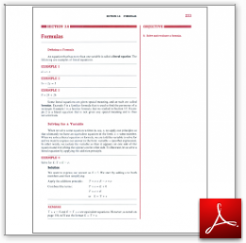Algebra Math Book - Introductory Algebra Thank you for your continual support.

 The power of mathematics rests in the logic of thinking.ID: Sec3-6
Description: Literal Equation-Solving for a Variable-Evaluating a Formula
Price: 1.99

# Algebra Math book - Introductory Algebra - Chapter 3 - Section 6 - Formulas

##Section 3.6 - Objectives

8.  Solve and evaluate a formula.

Navigate to
Previous Section or Next Section
or Chapter 3 Details or Other Chapters

This section of my algebra math book, Introductory Algebra, also includes in the download:

• Cover Sheet